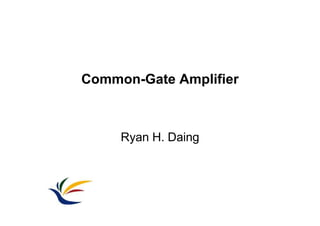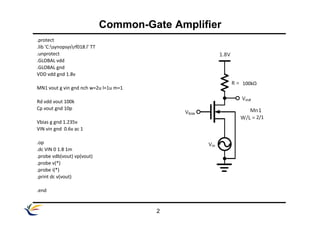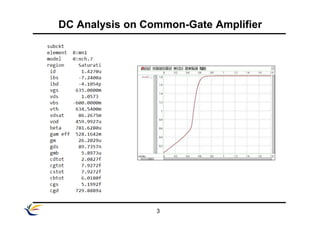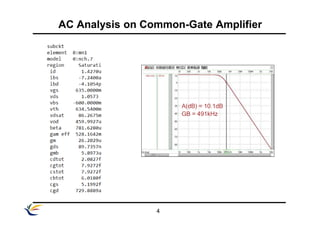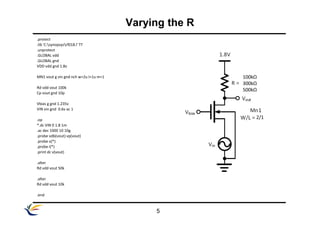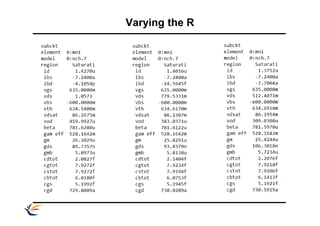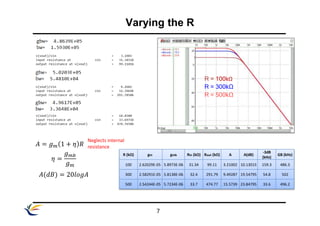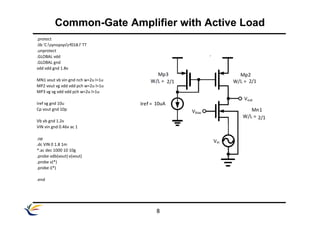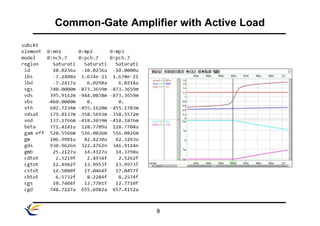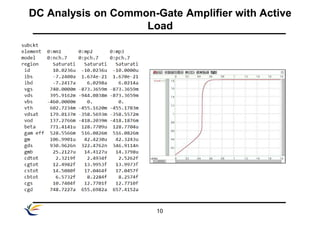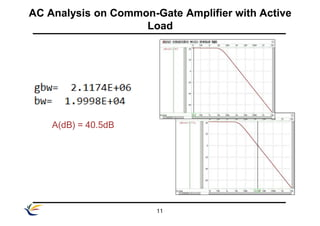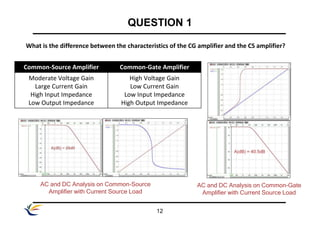1 of 12

### Common Gate Amplifier.pptx

1. Ryan H. Daing Common-Gate Amplifier
2. Common-Gate Amplifier 2 .protect .lib 'C:synopsysrf018.l' TT .unprotect .GLOBAL vdd .GLOBAL gnd VDD vdd gnd 1.8v MN1 vout g vin gnd nch w=2u l=1u m=1 Rd vdd vout 100k Cp vout gnd 10p Vbias g gnd 1.235v VIN vin gnd 0.6v ac 1 .op .dc VIN 0 1.8 1m .probe vdb(vout) vp(vout) .probe v(*) .probe i(*) .print dc v(vout) .end 100kΩ 2/1
3. 3 DC Analysis on Common-Gate Amplifier
4. 4 A(dB) = 10.1dB GB = 491kHz AC Analysis on Common-Gate Amplifier
5. 5 .protect .lib 'C:synopsysrf018.l' TT .unprotect .GLOBAL vdd .GLOBAL gnd VDD vdd gnd 1.8v MN1 vout g vin gnd nch w=2u l=1u m=1 Rd vdd vout 100k Cp vout gnd 10p Vbias g gnd 1.235v VIN vin gnd 0.6v ac 1 .op *.dc VIN 0 1.8 1m .ac dec 1000 10 10g .probe vdb(vout) vp(vout) .probe v(*) .probe i(*) .print dc v(vout) .alter Rd vdd vout 50k .alter Rd vdd vout 10k .end Varying the R 100kΩ 300kΩ 500kΩ 2/1
6. Varying the R
7. 7 𝐴 = 𝑔𝑚 1 + 𝜂 𝑅 𝐴(𝑑𝐵) = 20𝑙𝑜𝑔𝐴 R = 100kΩ R = 300kΩ R = 500kΩ 𝜂 = 𝑔𝑚𝑏 𝑔𝑚 Neglects internal resistance R (kΩ) gm gmb Rin (kΩ) Rout (kΩ) A A(dB) -3dB (kHz) GB (kHz) 100 2.62029E-05 5.8973E-06 31.34 99.11 3.21002 10.13015 159.3 486.3 300 2.58291E-05 5.8138E-06 32.4 291.79 9.49287 19.54795 54.8 502 500 2.54244E-05 5.7234E-06 33.7 474.77 15.5739 23.84795 33.6 496.2 Varying the R
8. 8 .protect .lib 'C:synopsysrf018.l' TT .unprotect .GLOBAL vdd .GLOBAL gnd vdd vdd gnd 1.8v MN1 vout vb vin gnd nch w=2u l=1u MP2 vout vg vdd vdd pch w=2u l=1u MP3 vg vg vdd vdd pch w=2u l=1u Iref vg gnd 10u Cp vout gnd 10p Vb vb gnd 1.2v VIN vin gnd 0.46v ac 1 .op .dc VIN 0 1.8 1m *.ac dec 1000 10 10g .probe vdb(vout) v(vout) .probe v(*) .probe i(*) .end Common-Gate Amplifier with Active Load 2/1 2/1 2/1 10uA
9. 9 Common-Gate Amplifier with Active Load
10. 10 DC Analysis on Common-Gate Amplifier with Active Load
11. 11 AC Analysis on Common-Gate Amplifier with Active Load A(dB) = 40.5dB
12. 12 QUESTION 1 What is the difference between the characteristics of the CG amplifier and the CS amplifier? Common-Source Amplifier Common-Gate Amplifier Moderate Voltage Gain Large Current Gain High Input Impedance Low Output Impedance High Voltage Gain Low Current Gain Low Input Impedance High Output Impedance A(dB) = 40.5dB AC and DC Analysis on Common-Source Amplifier with Current Source Load AC and DC Analysis on Common-Gate Amplifier with Current Source Load

### Editor's Notes

1. The code in the left was used to simulate the circuit on the right. The resistor used has a value of 100kΩ and the W/L ratio of the NMOS is 2/1.
2. Vbias >= 1.235V Saturation Vbias < 1.235V Cutoff The bias voltage at the gate terminal was 1.235V because a lesser value than that will make the NMOS in the Cutoff state.
3. The gain for the circuit was 10.1dB with a gain bandwidth of 491kHz.
4. In step 2, it was tasked to change the value of the resistor on the circuit. The resistance was decreased by 100kΩ, 300kΩ, and 500kΩ in order. Other parameters were left unchanged.
5. The condition of the NMOS while varying the resistor on the drain is shown in the following tables.
6. The hand calculated gain was somewhat similar to the simulated gain. As the resistance was increased, gain also increases and the bandwidths are decreasing.
7. A current mirror was used in order to simulate a current source load in the common-gate amplifier. The MOSFETs used has a W/L ratio of 2/1. The bias current used was 1.2V.
8. VIN > 0.46V MP2 becomes linear VIN < 0.45V MN1 becomes linear To ensure that the MOSFETs are in the saturation region, the VIN used was 0.46V. A VIN greater than 0.46V will make the MP2 to operate in the linear region. A VIN lesser that 0.45V will operate the MN1 in the linear region.
9. The Vout starts to saturate when the VIN is around 0.6V.
10. The gain of the circuit reached to around 40.5dB with a gain bandwidth of around 2.12MHz.